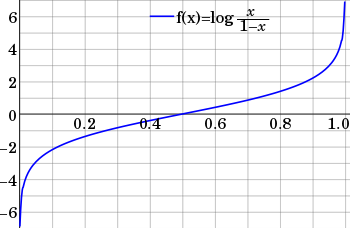# Logit

## What is a Logit?

A Logit function, also known as the log-odds function, is a function that represents probability values from 0 to 1, and negative infinity to infinity. The function is an inverse to the sigmoid function that limits values between 0 and 1 across the Y-axis, rather than the X-axis. Because the Logit function exists within the domain of 0 to 1, the function is most commonly used in understanding probabilities.Source

## How does a Logit function work?

The Logit function is represented as:
If X represents a probability, then X(1-X) is the odds, and the Logit function is the logarithm of the odds. The function plots across the graph within the domain of 0 to 1, and producing real numbers ranging from negative infinity to infinity.

### Logit Functions and Machine Learning

The Logit function is used similarly to the sigmoid function in neural networks. The sigmoid, or activation, function produces a probability, whereas the Logit function takes a probability and produces a real number between negative and positive infinity. Like the sigmoid function, Logit functions are often placed as the last layer in a neural network as can simplify the data. For example, a Logit function is often used in the final layer of a neural network used in classification tasks. As the network determines probabilities for classification, the Logit function can transform those probabilities to real numbers.